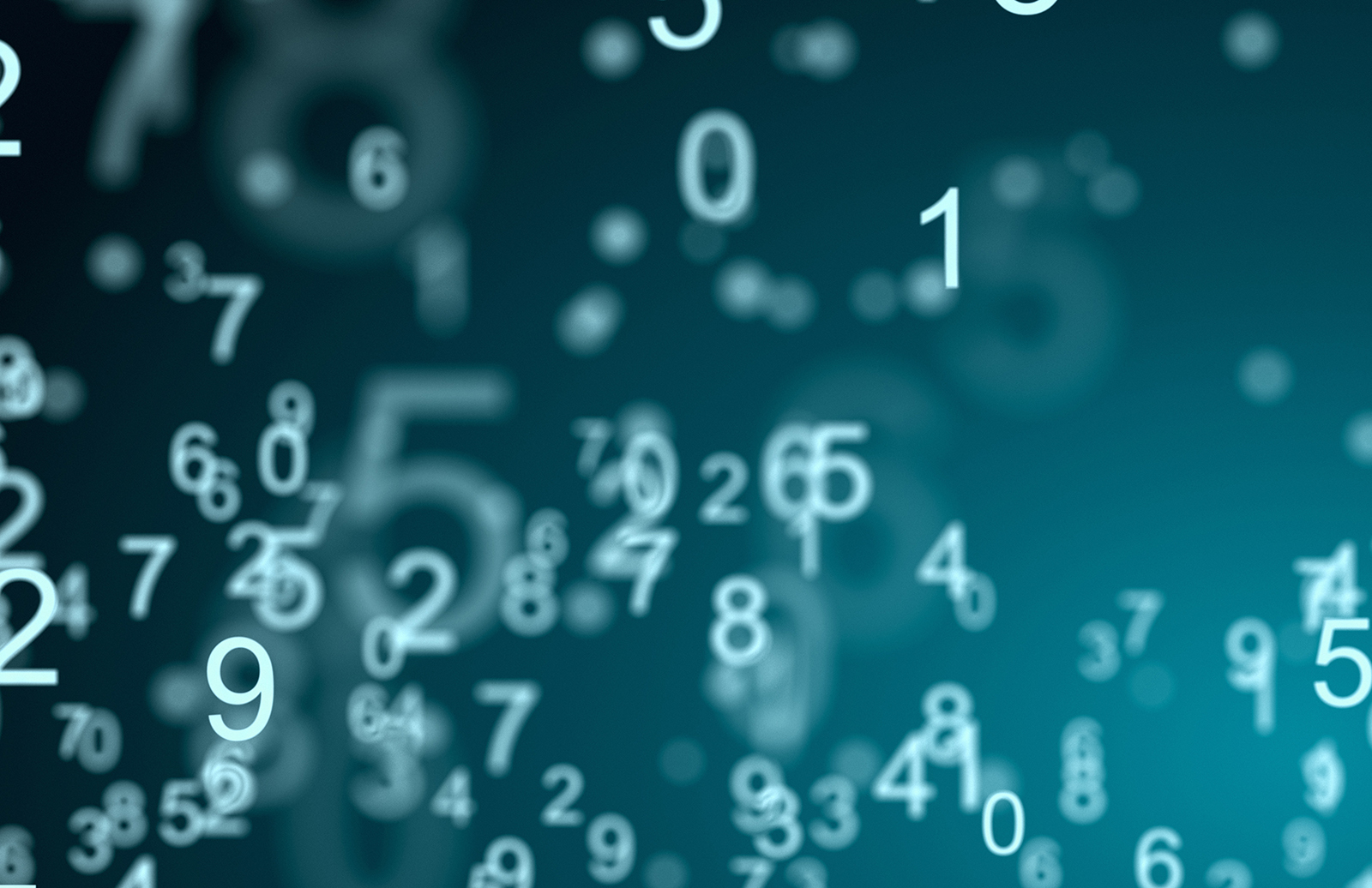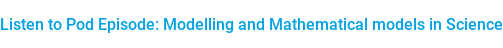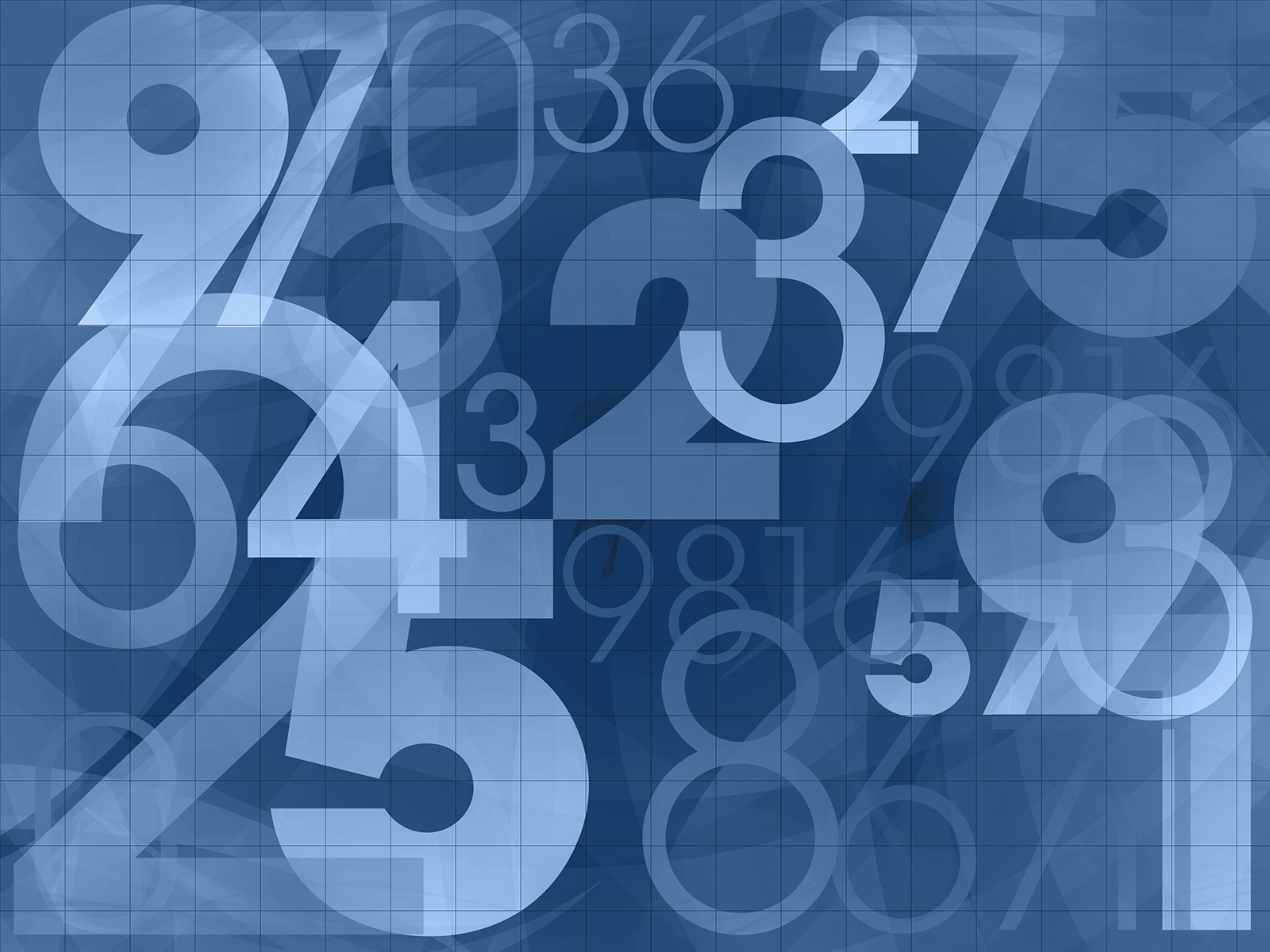Malin Edvardsson Apr 13, ’23 < 16 min

# A conversation on mathematical modelling in science

## Surface Science Blog

Modelling is a key component of science. So, what is modelling? What different types of models are there, and what is important to keep in mind when modelling? Curious to learn more, we talked to Prof. Marina Axelson-Fisk, Professor in Mathematical Statistics at Chalmers University of Technology, who shares some of her vast knowledge on mathematical modelling and how this is used in science.

## What is a mathematical model and what is modeling?

A mathematical model, or a model in general, is an abstract representation of some real-life object, Prof. Axelson-Fisk says. For example, a sculpture - that is a model of whatever it is portraying. And a painting is a model of a picture. The mathematical model is similar, but in formulas. So, we are representing things in the real world with formulas. Typically, you want to relate different factors, features, or entities in the real world and see how they relate. So that is a brief explanation of the model - it is an abstract version of reality, of different features in the reality. As such, it is of course, a simplification of the real world, Prof. Axelson-Fisk says.

The mathematical model represents a phenomenon or process and in the mathematical formula, you have variables representing the features or entities that you want to measure, Prof. Axelson-Fisk says. Then, you have parameters that are constants that adjust how the variables relate to each other. Those are the typical formulations in a in a mathematical model. The variable is what you measure, i.e., these are the unknowns that you want to understand more of.## When to use modelling

In science, you often want to either determine the relationship between features, i.e., you want to understand how different features in the real world relate to each other or affect each other, or you may want to understand how different features interact with each other, Prof. Axelson-Fisk says. So, the combination of features may have a different influence on whatever you measure compared to each of them separately. Another scenario is where you want to predict something. Say for example that you want to predict something that is difficult to measure but you have related entities that are easy to measure and that are connected. Then you make a mathematical formulation of how these entities connect to the thing you want to measure, Prof. Axelson-Fisk says.

This is very abstract, so let us take an example, for example predicting the weather. Typically, you predict the weather by looking at the weather history. The weather tomorrow is related in various ways to the weather today. In an advanced approach you could for example use satellite pictures which contain valuable information about historic weather, and these can be used as input in the model to predict the weather in the future, Prof. Axelson-Fisk says.

You use the model to predict things or to classify things, so, it can be used in many ways. In science, in applied science, you usually want to test out relationships in various ways using hypothesis testing, for instance. And then you need a model, Prof. Axelson-Fisk says.

## Different types of models - Linear vs non-linear

There are many different types of models, and they can be grouped into different categories. One important distinction is whether the model is linear or nonlinear. In a linear model, there is a linear relationship between the variables. If you only have two variables that relate to each other, you can draw a line. If you have more variables, then it becomes more complex, but you still have a linear relationship. This means that if you have more than one feature affecting the outcome of something, then the different features add up. So, you have the feature times a parameter constant and then you add them up.

In a nonlinear relationship on the other hand, you can have all kinds of functions. One example is the exponential function, i.e., ‘e’ to the power of your variable. The distinction between linear and nonlinear models is important because linear relationships are much easier to handle, both to train the model and to interpret it. In many applications, even though the relationship maybe is not exactly linear, you can often get away with approximating a linear relationship, Prof. Axelson-Fisk says.

### A phenomenon that can be described by a linear equation

As an example of a linear relation, I can mention my own master thesis, which I did for quite a while ago, Prof. Axelson-Fisk says. Then I made a model to estimate the age of fetuses. This would be useful in a situation where you have a pregnant woman that does not know exactly which week she is in and would like to estimate the age of the fetus. I made a model where the length of the femur and the diameter of the skull were used as input. We had several data points, i.e., several fetuses where we knew the age and corresponding values of the lengths of the femurs and diameters of the skulls. Then we related those parameters in a linear way to estimate the fetus’s age. When there was a new pregnant woman, we measured these parameters to estimate the age of the fetus. So that is an example of a linear relationship, Prof. Axelson-Fisk says.

## Another grouping of models - deterministic and stochastic, or random and nonrandom

In a deterministic model, all your variables are set. They can be unknown, but typically the same input will always yield the same output, Prof. Axelson-Fisk says. So, it is a fixed or constant relationship. In stochastic models, on the other hand, you have some addition of randomness. You may have some noise in your measurements, so you have a distribution over your variables, i.e., it is not an exact relationship. That is the main distinction. And then, instead of having measured variables, i.e., determined by the measurement, you also add on the randomness or the noise through distribution of random values.

## Discrete vs continuous models

The third important distinction is whether the model is discrete or continuous, i.e., the variables are discrete or continuous, Prof. Axelson-Fisk says. Typically, if you have a measurement device, you will get something that is continuous meaning that you can get any value between any two values. In a discrete model, however, you can separate the values and count them. They can be infinitely many, but you would still be able to count them, Prof. Axelson-Fisk says.

## How to create a model

So how do you create a model from scratch, or even just continue to work on something that exists but does not really describe the world in a good enough way? So, first you need data, Prof. Axelson-Fisk says. If you want to model the relationships, you need some data and then you need to see how they relate in various ways. The relationships can be explored by visualizing the data, plotting it in different ways. Then you would see, for instance, if it is a linear relationship or if there is some other pattern.

To start completely from scratch is difficult though. You need to have some idea of what relations and or phenomenon you are studying, so you need a lot of knowledge. Typically, you would also survey the field so you would investigate what different methods there are and pros and cons with those. And you need to determine whether you want a deterministic or stochastic model, whether it should be linear or nonlinear. You also need to consider that every model is simplification of the real world, Prof. Axelson-Fisk says. But there is a tradeoff between complexity and accuracy. The more complex your model is, the more accurate it is. But on the other hand, it will be much harder to handle, and you need a lot of data to train it. So, you must weigh the complexity towards the accuracy in some sense, Prof. Axelson-Fisk says.

You could also try to invent new models by combining existing ones. That is the kind of work I do a lot. I work typically on creating new models and we rarely develop them from scratch, but instead use existing models and tweak them or combine different parts, Prof. Axelson-Fisk says.

## Improving the model

To adjust the model, you have to have some sort of idea of what changes to make. If you see that something does not fit, then you need to figure out why it does not fit. For instance, if you are doing a linear regression model where you have an output and then you have one or several input variables, if you have done it as a linear relationship, then you can check whether this is a good assumption. And if it is not, then you might to want to change it to adapt your function data. If you see that there seem to be dependencies between the data, you need to take care of that. Maybe there are additional features that need to be included in the model and so on, Prof. Axelson-Fisk says.

## When is the model good enough?

There is a tradeoff between model complexity and accuracy, so where to draw the line in terms of trying to improve the model depends on what you are doing, Prof. Axelson-Fisk says. This could be considered already from the start - how big variation can I accept? If you have a stochastic model, it is about how big variation the data is. If the variation is too big, you need to either have a better model or have a larger dataset and so on. If you are training your model iteratively, for instance, then then you can for example have some error function that tells you how far from the truth you are when you train on annotated data. You can say that when I'm within this interval from that, then my parameter estimates are good enough and I am done. Or, when the error does not change between iterations, i.e., it becomes constant or near constant, then that would be a place to stop, because then it you cannot do it better, Prof. Axelson-Fisk says. If that is still not good enough, then you need to review your data and your model. This relates to the design of experiments that we talked about in a previous pod episode. You need to think things through and try to understand if there are variables that you cannot control which are still affecting your output. Then you need to figure out if you can solve that somehow. For instance, if you have some model where you measure things, and the temperature matters, then you may try to measure under the same temperature every time, under the same circumstances and so on. So, there are many things to think of and that you can tweak, but that that can also affect your results, Prof. Axelson-Fisk says.

## Mathematical modelling in science

Listen to the full interview with Prof. Axelson-Fisk to learn more about mathematical modelling, typical challenges and pitfalls to avoid.Podcast## Explore the blog

You have only scratched the surface.